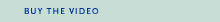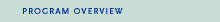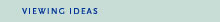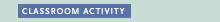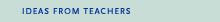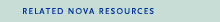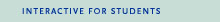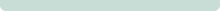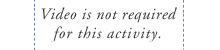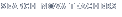Back to Teachers HomeProof, TheClassroom ActivityObjective
To explore simple curves.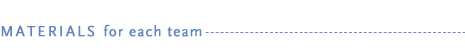• copy of "When is a Line Not a Line?" student handout (PDF or HTML)
• 1 sheet of graph paper with 1-inch grid squares
• ruler
• pencil with a sharp tip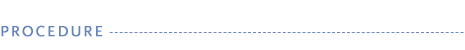1. Elliptic curves and modular forms were an essential part of Andrew Wiles' proof of Fermat's Last Theorem. Students can explore simple curves by constructing their own in this activity.

2. Gather materials and distribute "When Is a Line Not a Line?" student handout to students. (For best results, use graph paper with lines that are accurately printed.)

3. Before beginning the activity, introduce the term curve, and have students construct a definition with which all can agree. This definition may be challenged and revised as students start creating their designs.

4. Students will construct curves on graph paper by connecting a series of points between the vertical and horizontal axes.

5. After they have constructed their curves, have students share their designs and explain the patterns they notice among the segments that make up each curve. Did their definition of a curve change from when they first started the activity? If so, how?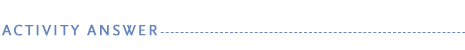Part I
Students will notice that a curved line is formed from the modules created by the intersection of the straight lines they drew between the vertical and horizontal axes. The curve is made up of many, small straight lines, called modules. The variation in length among the modules, along with a slight change of each module's position relative to each axis, creates the simple curve.

As students measure the length of each module, they will notice that the lengths differ in a regular pattern. (If a regular pattern does not emerge, have students recheck their grids for drawing errors.) By drawing a line through the 45 degree angle, students can observe that the curve is symmetric. A curve formed from an even number of draw points will result in an even number of modules on either side of the line of symmetry. A curve formed from an odd number of draw points will have one of its modules bisected by the line of symmetry.

Part II
When the distance between draw points is decreased, the resulting curve is made up of shorter modules and appears smooth. When the distance between draw points is increased, the resulting curve is made up of longer modules and appears choppy. Depending on whether there is an even number of draw points or an odd number of draw points along the vertical and horizontal axes, the module measurements will differ in regular ways for each. As an extension, students can experiment with changing the angle of the axes to create elliptic curves. Slightly wider curves will result when the angles are greater than 90 degrees; tighter curves will result when the angles are less than 90 degrees.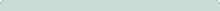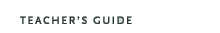Proof, The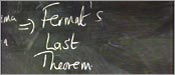Original broadcast:October 28, 1997